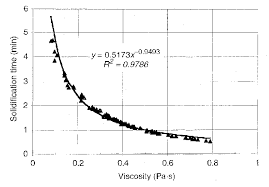## How to Calculate and Solve for Solidification Time | Solidification of MetalsThe image above represents solidification time.

To compute for solidification time, three essential parameters are needed and these parameters are Chvorinov’s Constant (C), Volume (V) and Surface Area (A).

The formula for calculating solidification time:

t = C(V / A)

Where:

t = Solidification Time
C = Chvorinov’s Constant
V = Volume
A = Surface Area

Let’s solve an example;
Find the solidification time when the chvorinov’s constant is 15, the volume is 12 and the surface area is 8.

This implies that;

C = Chvorinov’s Constant = 15
V = Volume = 12
A = Surface Area = 8

t = C(V / A)
t = 15(12 / 8)
t = 15(1.5)
t = 22.5

Therefore, the solidification time is 22.5s.

Calculating the Chvorinov’s Constant when the Solidification Time, the Volume and the Surface Area is Given.

C = tA / V

Where:

C = Chvorinov’s Constant
t = Solidification Time
V = Volume
A = Surface Area

Let’s solve an example;
Find the Chvorinov’s Constant when the solidification time is 20, the volume is 12 and the surface area is 8.

This implies that;

t = Solidification Time = 20
V = Volume = 12
A = Surface Area = 8

C = tA / V
C = (20)(8) / 12
C = 160 / 12
C = 13.3

Therefore, the chvorinov’s constant is 13.3